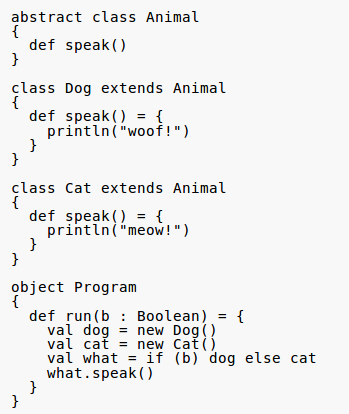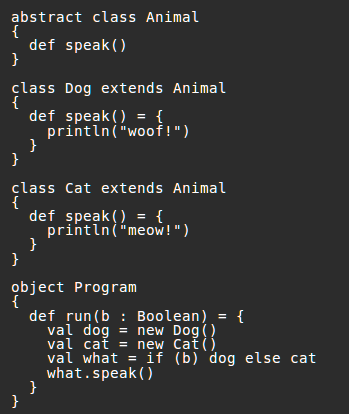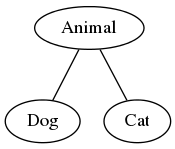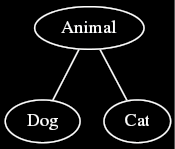# Join and meet: Examples

A union of sets and the least common multiple of a set of natural numbers can both be viewed as joins. In addition, joins can be useful to the designers of statically typed programming languages.

knows-requisite(Real analysis):

## The real numbers

Consider the partially ordered set $$\langle \mathbb{R}, \leq \rangle$$ of all real numbers ordered by the standard comparison relation. For any non-empty $$X \subseteq \mathbb{R}$$, $$\bigvee X$$ exists if and only if $$X$$ has an upper bound; this fact falls directly out of the definition of the set of real numbers. <div>

## Subtyping

Statically typed programming languages often define a poset of types ordered by the subtyping relation; Scala is one such language. Consider the following Scala program.When a programmer defines a class hierarchy in an object-oriented language, they are actually defining a poset of types. The above program defines the simple poset shown in the following Hasse diagram.comment: dot source:

digraph G { node = 0.1, height = 0.1; edge = “none”; rankdir = BT; Dog → Animal; Cat → Animal; } <div>

Now consider the expression if (b) dog else cat. If b is true, then it evaluates to a value of type Dog. If b is false, then it evaluates to a value of type Cat. What type, then, should if (b) dog else cat have? Its type should be the join of Dog and Cat, which is Animal.

## Power sets

Let $$X$$ be a set. Consider the partially ordered set $$\langle \mathcal{P}(X), \subseteq \rangle$$, the power set of $$X$$ ordered by inclusion. In this poset, joins are unions: for all $$A \subseteq \mathcal{P}(X)$$, $$\bigvee A = \bigcup A$$. This can be shown as follows. Let $$A \subseteq \mathcal{P}(X)$$. Then $$\bigcup A$$ is an upper bound of $$A$$ because a union contains each of its constituent sets. Furthermore, $$\bigcup A$$ is the least upper bound of $$A$$. For let $$Z$$ be an upper bound of $$A$$. Then $$x \in \bigcup A$$ implies $$x \in Y$$ for some $$Y \in A$$, and since $$Y \subseteq Z$$, we have $$x \in Y \subseteq Z$$. Since $$x \in \bigcup A$$ implies $$x \in Z$$, we have $$\bigcup A \subseteq Z$$. Hence, $$\bigvee A = \bigcup A$$.

## Divisibility

Consider the poset $$\langle \mathbb Z_+, | \rangle$$ of divisibility on the positive integers. In this poset, the upper bounds of an integer are exactly its multiples. Thus, the join of a set of positive integers in $$\langle \mathbb Z_+, | \rangle$$ is their least common multiple. Dually, the meet of a set of positive integers in $$\langle \mathbb Z_+, | \rangle$$ is their greatest common divisor.

• @1 This page includes a conditional example that only shows up for people who know real analysis. I’m imagining that someone who reads this may have real analysis familiarity, but has not marked themselves as such on Arbital. I’m not sure what the solution to this is. Maybe it is to automatically add a section at the bottom saying that there is additional content for people who know the subjects that are mentioned in conditionals.

• Good question! I’ll think about this, but your proposed solution sounds like a good start.

• Could you add an intro/​summary? It’s good to have for both popups and avoiding having two headers at the top.